This header plots the critical line of the Riemann Zeta Function.  A complete understanding wins a \$1,000,000 prize.
 . . .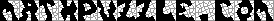Main Links Orders Post Next Page Next + 10Odds of a dimpled die, out of 10,000 tosses, using a Markov Chain model. This page contains a complete list of all possible Fair Dice.  First off, objects which are ISOHEDRAL are fair.  To be isohedral, each face must have the same relationship with all other faces, and each face must have the same relationship with the center of gravity.  Thus, each object has a Group structure (a Group Theory topic).  Using Group Theory and Euler's formula (Vertices + Faces - Edges = 2), it can be proven that the following list contains all possible isohedra.  An interactive version of this page is at the CRC Concise Encyclopedia of Mathematics.  A good explanation of the math behind all this can be found at Klaus Æ. Mogensen's website.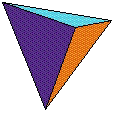Regular TetrahedronIsosceles Tetrahedron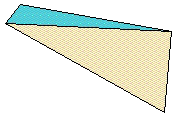Scalene Tetrahedron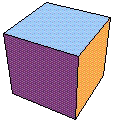Cube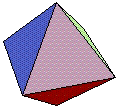OctahedronRegular DodecahedronOctahedral Pentagonal Dodecahedron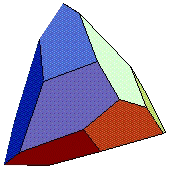Tetragonal Pentagonal Dodecahedron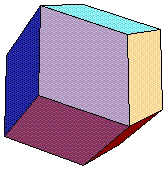Rhombic Dodecahedron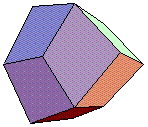Trapezoidal Dodecahedron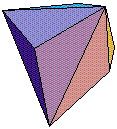Triakis Tetrahedron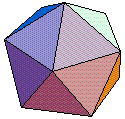Regular IcosahedronHexakis TetrahedronTetrakis HexahedronTriakis Octahedron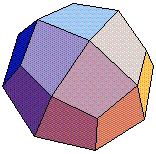Trapezoidal Icositetrahedron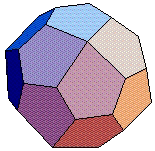Pentagonal Icositetrahedron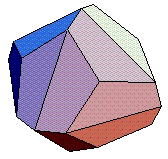Dyakis Dodecahedron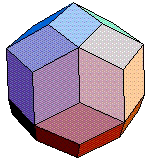Rhombic Triacontahedron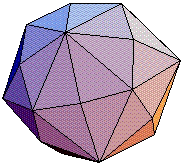Hexakis OctahedronTriakis IcosahedronPentakis Dodecahedron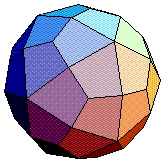Trapezoidal Hexecontahedron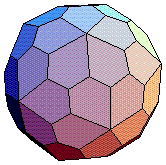Pentagonal HexecontahedronHexakis Icosahedron 120 sidesTriangular Dihedron Move points up/down - 4N sides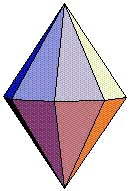Basic Triangular Dihedron 2N sidesTrigonal Trapezohedron Asymmetrical sides -- 2N SidesBasic Trigonal Trapezohedron Sides have symmetry -- 2N SidesTriangular Dihedron Move points in/out - 4N sides

Note to gaming companies:  I'd love to see someone make the exotic dice in the list above. It would be the best gambling game since King Solomons slots. Imagine the odds.

Can a non-isohedral fair die exist?  Consider a pyramid made from 4 isosceles triangles and a square.  If the pyramid is short and fat, the square face will be landed upon more than a fifth of the time.  If the pyramid is tall and thin the square face will be landed upon less than a fifth of the time.  Is there a height where the square face will be landed upon exactly one fifth of the time??? Yes, for a given set of conditions.  If you knew the height, force, elasticity, and throwing method, you could find the right height.  However, once the conditions changed, the die would no longer be fair.  (NOTE:  I have a strong argument for this, but no proof.)

It is important to fully understand the details behind the games we play and the odds and probabilities. Now that you can play dice game online understanding the odds becomes a software issue. There is a big difference between playing online roulette and roulette at a table. Either way by studying the game you will always get the best results.

If you find the polyhedrons above interesting, you're bound to enjoy the Pavilion of Polyhedreality by George Hart.

There are 6 regular 4-dimensional object.  Peek is a fascinating and very pretty piece of software that allows looking at 3-D cross-sections of complex 4-D objects.  The pictures are beautiful!